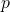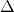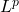# Convergence theorem for an intermixed iteration in p-uniformly convex metric space

### Full PDFcarpathian_2023_39_2_459_475

In this paper, we first introduce the intermixed algorithm in-uniformly convex metric spaces, and then we prove-convergence of the proposed iterative method for finding a common element of the sets of fixed points of finite families of nonexpansive mappings in the framework of complete-uniformly convex metric spaces. Furthermore, we apply our main theorem to prove-convergence to solve the minimization problems in the framework of complete-uniformly convex metric spaces. Finally, we give two examples inspaces and numerical examples to support our main results.